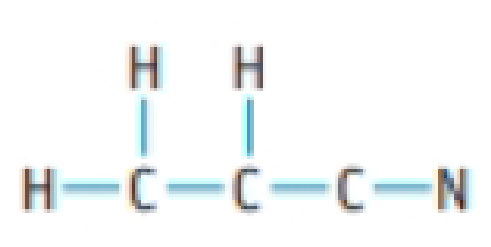Chapter 8, Problem 8PS

Chapter
Section
Textbook Problem

Draw a Lewis structure for each of the following molecules: (a) methanol, CH3OH (b) vinyl chloride, H2C=CHCl, the molecule from which PVC plastics are made (c) acrylonitrile, H2C=CHCN, the molecule from which materials such as Orion are made(a)

Interpretation Introduction

Interpretation: It should be drawn the Lewis structure of the given following molecules.

Concept Introduction:

• Lewis structures are diagrams that represent the chemical bonding of covalent molecules and coordination compounds.
• It is also known as Lewis dot structures which represent the bonding between atoms of a molecule and the lone pairs of electrons that may exist in the molecule.
• While drawing the skeletal structure, the least electronegative atom is considered as the central atom.
• If the more electronegative atom was placed as the central atom, then the concentration of negative charges becomes high which leads to the instability of the molecule.
Explanation

Lewis structure of methanol CH3OH

(b)

Interpretation Introduction

Interpretation: It should be drawn the Lewis structure of the given following molecules.

Concept Introduction:

• Lewis structures are diagrams that represent the chemical bonding of covalent molecules and coordination compounds.
• It is also known as Lewis dot structures which represent the bonding between atoms of a molecule and the lone pairs of electrons that may exist in the molecule.
• While drawing the skeletal structure, the least electronegative atom is considered as the central atom.
• If the more electronegative atom was placed as the central atom, then the concentration of negative charges becomes high which leads to the instability of the molecule.

(c)

Interpretation Introduction

Interpretation: It should be drawn the Lewis structure of the given following molecules.

Concept Introduction:

• Lewis structures are diagrams that represent the chemical bonding of covalent molecules and coordination compounds.
• It is also known as Lewis dot structures which represent the bonding between atoms of a molecule and the lone pairs of electrons that may exist in the molecule.
• While drawing the skeletal structure, the least electronegative atom is considered as the central atom.
• If the more electronegative atom was placed as the central atom, then the concentration of negative charges becomes high which leads to the instability of the molecule.

Still sussing out bartleby?

Check out a sample textbook solution.

See a sample solution

The Solution to Your Study Problems

Bartleby provides explanations to thousands of textbook problems written by our experts, many with advanced degrees!

Get Started

Today, an estimated 69 percent of the adults in the United States are overweight or obese.

Nutrition: Concepts and Controversies - Standalone book (MindTap Course List)

How does an ionic bond form?

Biology: The Dynamic Science (MindTap Course List)

Explain why fatty acids cannot serve as fuel for the brain.

Chemistry for Today: General, Organic, and Biochemistry